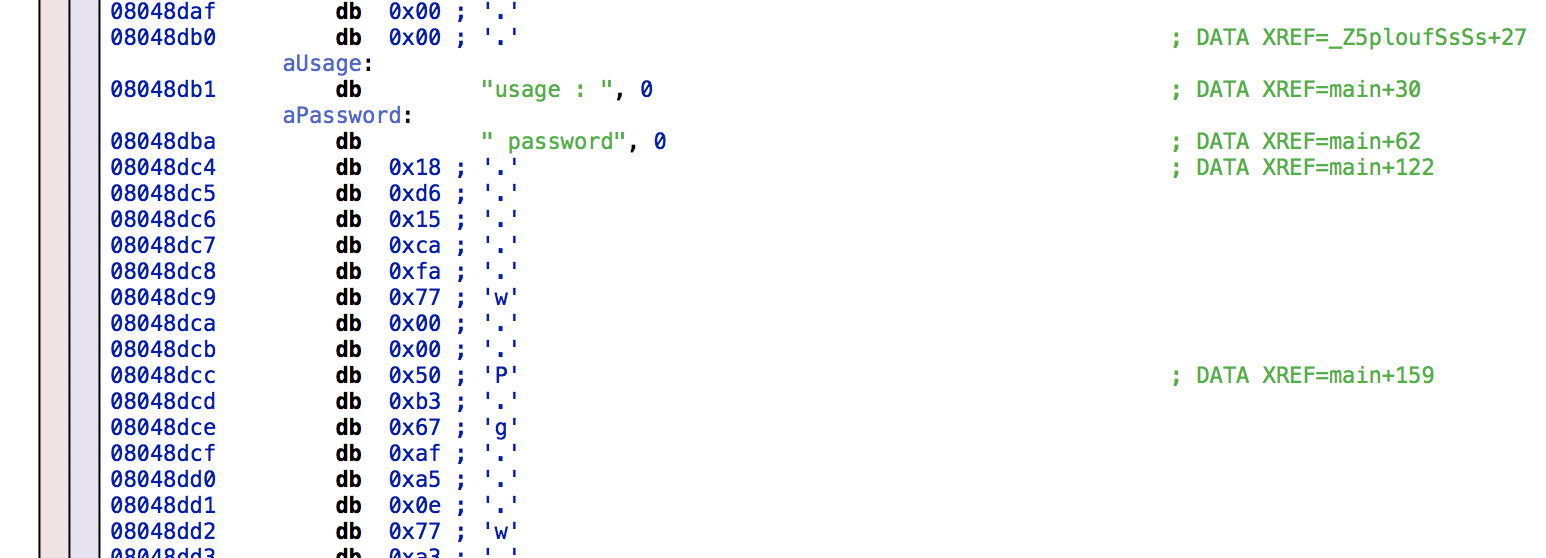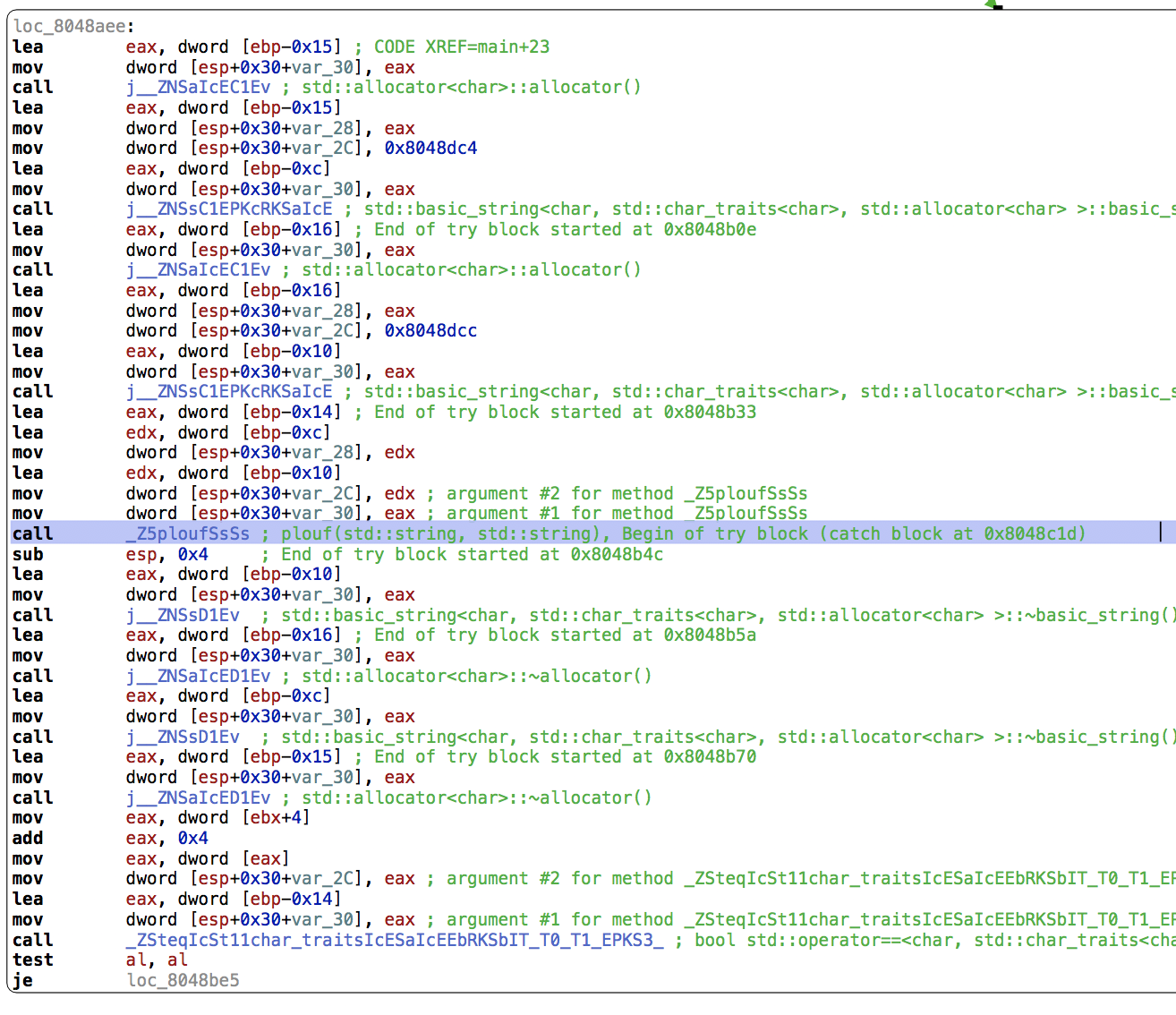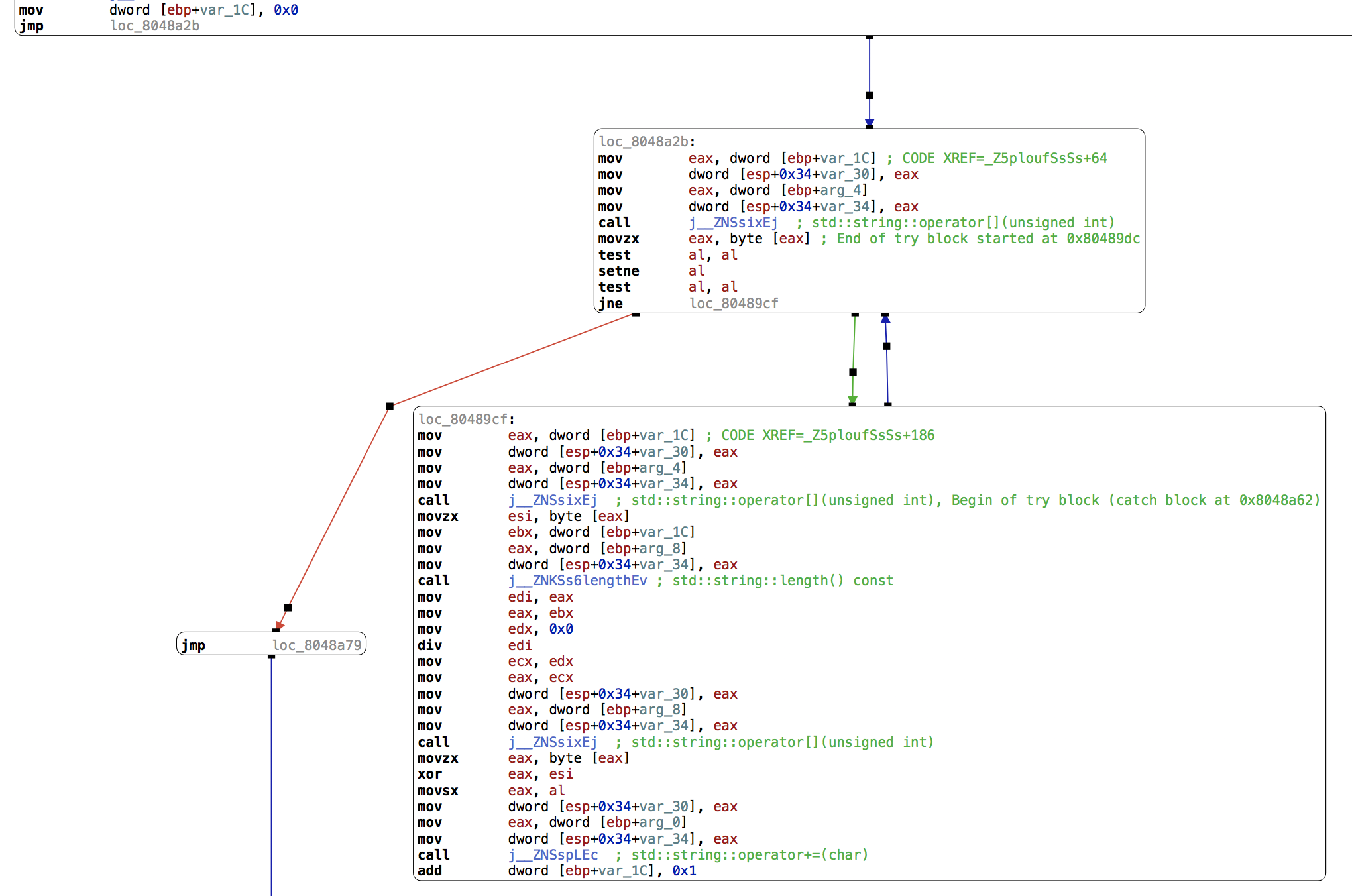# Crackme 4: ELF C++, no protection

## Mar 13, 2018

Our first C++ binary!

``````\$ ./ch25.bin
``````

Again, from strings we can find references to a password:`main` is much noisier with the many steps needed to construct a string object:Still, we can find a reference to the function `plouf(std::string, std::string)` which takes two strings constructed from buffers at addresses 0x8048dc4 and 0x8048dcc. Their contents are, respectively [0x18, 0xd6, 0x15, 0xca, 0xfa, 0x77] and [0x50, 0xb3, 0x67, 0xaf, 0xa5, 0x0e, 0x77, 0xa3, 0x4a, 0xa2, 0x9b, 0x01, 0x7d, 0x89, 0x61, 0xa5, 0xa5, 0x02, 0x76, 0xb2, 0x70, 0xb8, 0x89, 0x03, 0x79, 0xb8, 0x71, 0x95, 0x9b, 0x28, 0x74, 0xbf, 0x61, 0xbe, 0x96, 0x12, 0x47, 0x95, 0x3e, 0xe1, 0xa5, 0x04, 0x6c, 0xa3, 0x73, 0xac, 0x89]. They both end with a null byte, omitted here.

The `plouf` function:Let’s dig into it. `arg_4` is the first parameter to the function, and `arg_8` is the second. `arg_0` is our return value. `var_1C` is a counter, first initialized to zero:

``````mov        dword [ebp+var_1C], 0x0
jmp        loc_8048a2b
``````

Here, we call `operator[]` on `arg_4` with parameter `var_1C` and store the result in `eax`:

``````loc_8048a2b:
mov        eax, dword [ebp+var_1C] ; CODE XREF=_Z5ploufSsSs+64
mov        dword [esp+0x34+var_30], eax
mov        eax, dword [ebp+arg_4]
mov        dword [esp+0x34+var_34], eax
call       j__ZNSsixEj  ; std::string::operator[](unsigned int)
movzx      eax, byte [eax] ; End of try block started at 0x80489dc
``````

We check whether this value is zero:

``````test       al, al
setne      al
test       al, al
jne        loc_80489cf
``````

If it’s not zero, we continue below and copy `var_1C` into `eax`:

``````loc_80489cf:
mov        eax, dword [ebp+var_1C] ; CODE XREF=_Z5ploufSsSs+186
``````

We then call `operator[]` on `arg_4` at offset `eax`, and save the value in `esi`:

``````mov        dword [esp+0x34+var_30], eax
mov        eax, dword [ebp+arg_4]
mov        dword [esp+0x34+var_34], eax
call       j__ZNSsixEj  ; std::string::operator[](unsigned int), Begin of try block (catch block at 0x8048a62)
movzx      esi, byte [eax]
``````

We store our counter `var_1C` into `ebx`:

``````mov        ebx, dword [ebp+var_1C]
``````

Then call `string::length()` on `arg_8`:

``````mov        eax, dword [ebp+arg_8]
mov        dword [esp+0x34+var_34], eax
call       j__ZNKSs6lengthEv ; std::string::length() const
``````

We now have the length of `arg_8` in `eax`, we store it in `edi` and divide `ebx` by `edi`:

``````mov        edi, eax
mov        eax, ebx
mov        edx, 0x0
div        edi
``````

The remainder of this division is in `edx` (so `strlen(arg_8) % counter`), which we copy to `eax` via `ecx`:

``````mov        ecx, edx
mov        eax, ecx
``````

We call `operator[]` on `arg_8` with the parameter `eax` and store the value in `eax`:

``````mov        dword [esp+0x34+var_30], eax
mov        eax, dword [ebp+arg_8]
mov        dword [esp+0x34+var_34], eax
call       j__ZNSsixEj  ; std::string::operator[](unsigned int)
movzx      eax, byte [eax]
``````

We then XOR `eax` and `esi` and store the result in `eax` before appending it to `arg_0`:

``````xor        eax, esi
movsx      eax, al

mov        dword [esp+0x34+var_30], eax
mov        eax, dword [ebp+arg_0]
mov        dword [esp+0x34+var_34], eax
call       j__ZNSspLEc  ; std::string::operator+=(char)
``````

Finally, we increment our counter, `var_1C`:

``````add        dword [ebp+var_1C], 0x1
``````

The blue arrow means we’re jumping back to the previous block unconditionally.

We can reconstruct the code:

``````string plouf(string key, string input) {
string out = "";
int i = 0;

while (input[i] != 0) {
char input_char = input[i];
char key_char = key[i % key.length()];
char out_char = input_char ^ key_char;
out += out_char;
i++;
}

return out;
}
``````

A Python version:

``````>>> input = [0x50, 0xb3, 0x67, 0xaf, 0xa5, 0x0e, 0x77, 0xa3, 0x4a, 0xa2, 0x9b, 0x01, 0x7d, 0x89, 0x61, 0xa5, 0xa5, 0x02, 0x76, 0xb2, 0x70, 0xb8, 0x89, 0x03, 0x79, 0xb8, 0x71, 0x95, 0x9b, 0x28, 0x74, 0xbf, 0x61, 0xbe, 0x96, 0x12, 0x47, 0x95, 0x3e, 0xe1, 0xa5, 0x04, 0x6c, 0xa3, 0x73, 0xac, 0x89]
>>> key =  [0x18, 0xd6, 0x15, 0xca, 0xfa, 0x77]
>>> ''.join(map(chr, [input[i] ^ key[i % len(key)] for i in range(len(input))]))
'Here_you_have_to_understand_a_little_C++_stuffs'
``````

Let’s try it:

``````\$ ./ch25.bin Here_you_have_to_understand_a_little_C++_stuffs
Bravo, tu peux valider en utilisant ce mot de passe...
Congratz. You can validate with this password...
``````